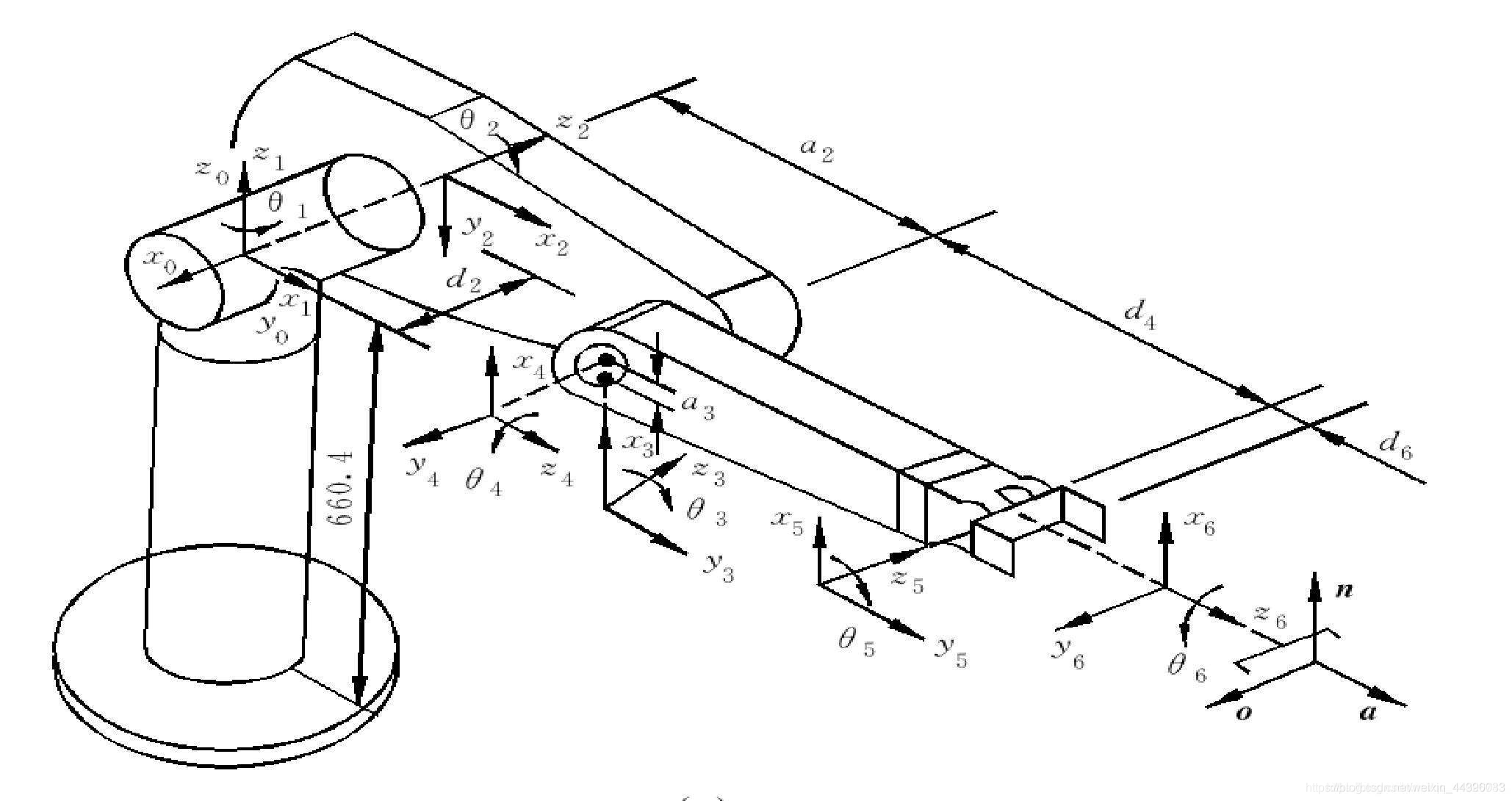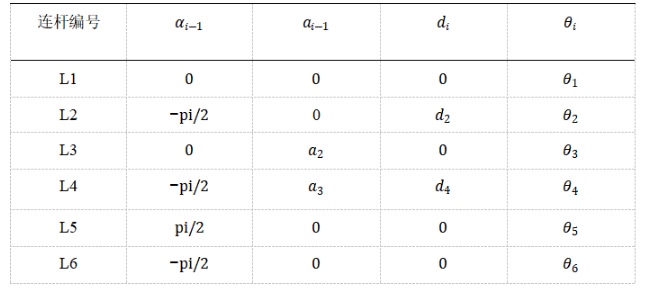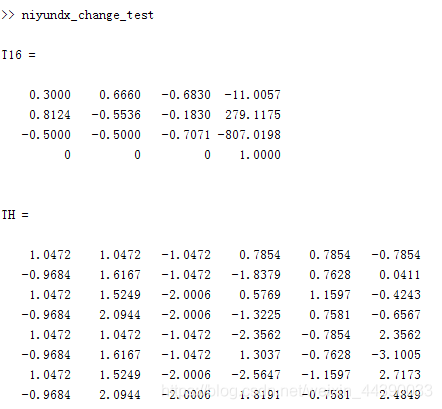• 阅读数：134发布于2021-07-16 19:27:26

只看该作者
###### 机械臂建模，轨迹规划，避障路径规划（介绍+代码）（一） 复制本文链接

最近写的一个作业，记录分享一下，主要是对机械臂在MATLAB中的仿真，其中包括机械臂建模，机械臂轨迹规划，机械臂避障路径规划等。
机械臂建模
机械臂建模一般采用dh建模方法，dh建模分为标准dh和改进dh，两种建模方法的参数表不同，适用的场景有些差异，具体可以看PUMA560机器人D-H参数和改进DH参数，这个是他人的博客，感觉写的很清楚，可以看一下。
本次是对PUMA560进行建模，使用的是改进dh建模方法，坐标系和dh参数表如下：实现方式有很多，以下介绍使用MATLAB中的robotics toolbox实现和使用改进dh转换矩阵进行实现：
robotics toolbox实现：

``````// modified DH
clear;
clc;
%建立机器人模型
% theta d a alpha offset
L1=Link([0 0 0 0 0 ],'modified'); %连杆的D-H参数
L2=Link([0 149.09 0 -pi/2 0 ],'modified');
L3=Link([0 0 431.8 0 0 ],'modified');
L4=Link([0 433.07 20.32 -pi/2 0 ],'modified');
L5=Link([0 0 0 pi/2 0 ],'modified');
L6=Link([0 0 0 -pi/2 0 ],'modified');
robot=SerialLink([L1 L2 L3 L4 L5 L6],'name','puma560','base' , ...
transl(0, 0, 0.62)* trotz(0)); %连接连杆，机器人取名puma560
robot.teach()
robot.display(); %显示D-H表
``````

转换矩阵实现：

``````// Transformation matrix
clear;
clc;

a1 = 0;ar1 = 0;d1 = 0;th1 = 0;
a2 = 0;ar2 = -pi/2;d2 = 149.09;th2 = pi/2;
a3 = 431.8;ar3 = 0;d3 = 0;th3 = 0;
a4 = 20.32;ar4 = -pi/2;d4 = 433.07;th4 = 0;
a5 = 0;ar5 = pi/2;d5 = 0;th5 = pi/2;
a6 = 0;ar6 = -pi/2;d6 = 0;th6 = 0;

T16 = eye(4);

for i=1:6
t1 = eval(['th' num2str(i)]);
t2 = eval(['ar' num2str(i)]);
t3 = eval(['d' num2str(i)]);
t4 = eval(['a' num2str(i)]);

T(:,:,i)=[cos(t1),-sin(t1),0,t4;
sin(t1)*cos(t2),cos(t1)*cos(t2),-sin(t2),-t3*sin(t2);
sin(t1)*sin(t2),cos(t1)*sin(t2),cos(t2),t3*cos(t2);
0,0,0,1];
end

for k=1:6
T16 = T16*T(:,:,k);
end

T16
``````

正逆运动学
正运动学可以直接使用以上的转换矩阵程序，也可以使用工具箱内fkine函数求解。由于工具箱的逆解函数只能得到一组解，在之后的避障路径规划时进行碰撞检测时需要所有可能解，则编写了逆运动学求解函数，该函数以PUMA560为例，输入为机械臂末端位姿，输出为机械臂八组逆解，程序下载见底部。以下为仿真结果：以上为输入关节角为th1=pi/3,th2=pi/3,th3=-pi/3,th4=pi/4,th5=pi/4,th6=-pi/4,情况下的仿真结果，正运动学的转换矩阵为T16，八组关节角为TH，可知第一组关节角即为输入的关节角度。

举报

• 垃圾广告
• 违规内容
• 恶意灌水
• 重复发帖